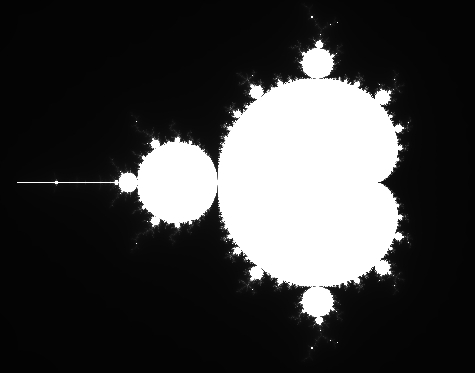CPSC 170 Post Lab 7Mandelbrot SetDue Before Class on Monday, March 8thThe Mandelbrot set is a set of complex numbers that remains bounded (does not increase to infinity) when iterated with a quadratic polynomial function. When the set is graphed on the complex plane the set boundary has a complex pattern that does not simplify at any magnification and is therefore sometimes defined as a fractal. Images of the Mandelbrot set boundary are common due to the simplicity of their creation and their beauty.

Details

The quadratic polynomial function can be defined recursively as:

cn+1 = cn2 + c0

where ci is a complex number. Recall that a complex number is a number that can be written with the form a + bi, where a and b are real values and i is √-1.

In order to determine whether a complex number remains bounded could potentially take an infinite amount of time. Instead, we can estimate whether a number is bounded by testing if it is bounded by a small number in a small number of iterations. For this assignment, assume that if the absolute value of ci, where 0 > i ≤ 255, is greater than 2, then c0 is not in the Mandelbrot set. If all ci, where 0 > i ≤ 255, are less than or equal to 2, then assume that c0 is in the Mandelbrot set.

In order to calculate whether some c0 is in the Mandelbrot set you should create a class that represents complex numbers. It will need instance variables for the real and complex parts of a number, as well as methods for addition, multiplication, and absolute value. Two complex numbers are multiplied using the equation:

(a + bi)(c + di) = (ac - bd) + (bc + ad)i

Two complex numbers are added using the equation:

(a + bi) + (c + di) = (a + c) + (b + d)i

The absolute value of a complex number is computed using:

|a + bi| = √(a2+b2)

Create a program that plots the Mandelbrot set for complex numbers in the range -2 > a, b < 2. To do this create a method or constructor in the complex number class that can convert the coordinates of a pixel in the window to a complex number in the specified range. For example, if the window is 200 by 200 pixels, the pixel (0, 0) would map to the complex coordinate (-2, -2) and the pixel (100, 100) to the complex coordinate (0, 0). Also create a method in the complex number class that returns the number of iterations of the quadratic polynomial function that are required to determine if a complex number is in the Mandelbrot set.

If a complex number is in the Mandelbrot set, draw the pixel as white, if it is not, draw it as a shade of gray depending on how many iterations of the quadratic polynomial function were required to determine that it is not in the set. For example, if the quadratic polynomial function is iterated 100 times before the absolute value becomes larger than 2, then draw the pixel with the RGB values (100, 100, 100). Each pixel can be drawn by using the fillRect method with a rectangle that is 1 by 1.

When the user clicks on the image have the image zoom in on the click location by changing the range of the complex numbers. For example, if the window is 200 by 200 and the complex range is halved on user clicks, a click on the pixel (100, 100) would set the complex range to -1 > a, b < 1. A click on the pixel (50, 50) would set the complex range to -2 > a, b < 0.

Lastly, zoom in on a portion of the image that creates an image that you like. Take a screen shot of the window and include it with your code submission.

Submission: Tar and submit your code on the course blackboard site.

Extra

Color Map

It is possible to create more visually pleasing images by using a color map to specify what color maps to which escape value. It is possible, for example, to have a color map between colors with RGB values (100, 255, 150) and (200, 50, 0) by interpolating between the two colors based on the escape value. It is possible to interpolate by using the equation from lab, but use it with each of the color channels. For example, if the escape value is 128, then the interpolated color of the above two colors would be r = (100 * (1 - i)) + (200 * i), g = (255 * (1 - i)) + (50 * i), b = (150 * (1 - i)) + (0 * i), where i = 128 / 255.

It is also possible to produce a color map with more than two colors by first determining which pair of colors an escape value is between, and then interpolating the colors. For example, if a color map consists of three colors and the escape value is 42, then because 42 / 255 is less than 0.5 the interpolated color would be produced by interpolating between the first and second colors with i = 42 / 127.

Julia Set

Julia sets are sets of complex numbers that are related to the Mandelbrot set and are also capable of producing interesting images. A complex number is determined to be in a Julia set in a similar fashion as for the Mandelbrot set. The difference is that the quadratic polynomial equation for a Julia set is:

cn+1 = cn2 + cj

Where cj is any complex number. The more interesting Julia sets correspond to complex numbers that are near the boundary of the Mandelbrot set. Some interesting values for cj you could try are 0.4+0.6i, 0.285+0.01i, and -0.835-0.2321i